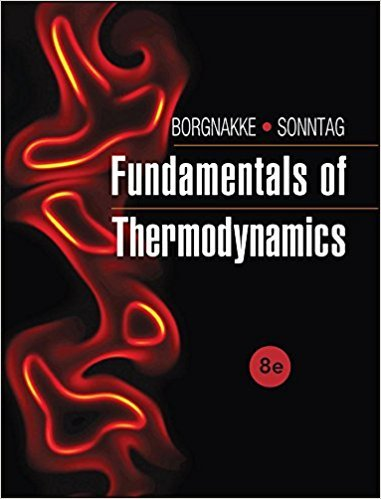×
Get Full Access to Fundamentals Of Thermodynamcs - 8 Edition - Chapter 3 - Problem 128hp
Get Full Access to Fundamentals Of Thermodynamcs - 8 Edition - Chapter 3 - Problem 128hp

×

# Use the ideal-gas air Table A.7 to evaluate the heatISBN: 9781118131992 58

## Solution for problem 128HP Chapter 3

Fundamentals of Thermodynamcs | 8th Edition

• Textbook Solutions
• 2901 Step-by-step solutions solved by professors and subject experts
• Get 24/7 help from StudySoup virtual teaching assistantsFundamentals of Thermodynamcs | 8th Edition

4 5 1 234 Reviews
16
1
Problem 128HP

Use the ideal-gas air Table A.7 to evaluate the heat capacity CP at 300 K as a slope of the curve h(T) by Δh/ΔT. How much larger is it at 1000 K and at 1500 K?

Step-by-Step Solution:

Solution 128HP

Step 1 of 3:

We are going to find the heat capacity Cp of the ideal gas for the given temperature and to compare the increase with respect to temperatures 1000K and 1500K.

The first temperature T = 300 K

The temperature values are taken from the table A.7 near the temperature T.

T1 = 320 K

T2 = 290 K

The specific enthalpies are given for the temperatures T1 and T2

h1 = 290.43 kJ/kg

h2 = 320.58 kJ/kg

The specific heat can be calculated from the slope of the h-T curve at T.Putting the valuesThe heat capacity of the ideal gas at 300 K is 1.0049 kJ/kg.K

Step 2 of 3

Step 3 of 3

##### ISBN: 9781118131992

This textbook survival guide was created for the textbook: Fundamentals of Thermodynamcs , edition: 8. The answer to “?Use the ideal-gas air Table A.7 to evaluate the heat capacity CP at 300 K as a slope of the curve h(T) by ?h/?T. How much larger is it at 1000 K and at 1500 K?” is broken down into a number of easy to follow steps, and 36 words. Since the solution to 128HP from 3 chapter was answered, more than 492 students have viewed the full step-by-step answer. Fundamentals of Thermodynamcs was written by and is associated to the ISBN: 9781118131992. This full solution covers the following key subjects: Table, pressure, relative, air, ideal. This expansive textbook survival guide covers 7 chapters, and 1462 solutions. The full step-by-step solution to problem: 128HP from chapter: 3 was answered by , our top Engineering and Tech solution expert on 08/03/17, 05:05AM.

Unlock Textbook Solution